Deutsche VersionThermal noise, Johnson noise,
Nyquist noise, and white noise

Calculation of Noise voltage
Noise voltage in microvolts (RMS), noise level in dBu, and dBV
signal-to-noise-ratio − Hi-Fi bandwidth from 20 Hz to 20 000 Hz = 19 980 Hz

 In common use, the word noise means unwanted sound or noise pollution. In electronics noise refers to the electronic signal in an audio system. Thermal noise is generated by thermal agitation of electrons in a conductor. A resistor makes noise without any voltage applied to it. Forget the power!

 Temperature ϑ ° Celsius Used bandwidth Δ f Hz Resistance R ohms ↓ RMS Noise voltage Vn μV (microvolts) Noise level Lu dBu Noise level LV dBV
 Formula for the RMS noise voltage: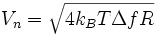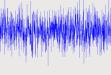The noise level Lu has the reference voltage V0 = 0.7746 V ≡ 0 dBu and the noise level LV has the reference voltage V0 = 1 V ≡ 0 dBV.

 Boltzmann constant kB = 1.3806504×10-23 J/K (joule/kelvin); J = W · s Absolute temperature in kelvin T = 273.15 + ϑ  in °C Bandwidth being considered Δ f = f2 − f1 = fmax − fmin in Hz; 20 kHz − 20 Hz = 19980 Hz Resistance of the circuit element R. R does not mean the universal gas constant!

To each noise potential the temperature T and the bandwidth Δ f
must be indicated, with which it was measured.

 To use the calculator, simply enter a value. The calculator works in both directions of the ↔ sign.

 Noise voltage level (audio) Lu dBu ↔ Noise voltage (audio) V  µV (microvolt)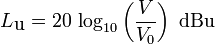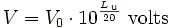Reference voltage V0 = 0.7746 Volt = 774 596.67 µV ≡ 0 dBu
 Noise voltage level LV dBV ↔ Noise voltage V  µV (microvolt)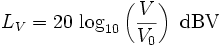Reference voltage V0 = 1.0 Volt = 1 000 000 µV ≡ 0 dBV

Don't forget the minus sign, when you enter the noise voltage level.

 Sound engineers or technicians with good ears care less about noise power or energy. That's OK. What does move our eardrums? What does move the diaphragm or membrane of a microphone of a sound pressure level meter (SPL meter)? Try to use less calculated sound power (acoustic power). Microphone developers, console developers and sound engineers are mostly interested in knowing the disturbing noise as voltage (!) at the output of the audio devices. Forget the power! The noise voltage can be measured. The noise power must be calculated.

 Note: The radiated sound power (sound intensity) is the cause and the sound pressure is the effect, where the sound engineer is particularly interested in the effect. The effect of temperature and sound pressure: Effect and Cause – Sound pressure and Sound power .

 Acousticians and sound protectors ("noise fighters") need the sound intensity ("acoustic intensity") – but sound engineers and sound designers ("ear people") don't need that sound energy quantity.   Who is involved in audio engineering, should rather take care of the sound field quantity, that is the sound pressure or the sound pressure level (SPL) as an effect at the eardrums of our hearing and on the diaphragms of the microphones, and the corresponding audio voltage and its voltage level.

Effect and Cause − Sound Pressure and Sound Power

Noise fighters (noise protectors) think differently. This is a special sound engineering site.
When you think of avoiding reflections by impedance matching (power matching) with Zin = Zout
that has only to do with high frequency RF, and not with audio.

White Noise Generator

 Thermal noise: Also called Johnson noise, is the random white noise generated by thermal agitation of electrons in a conductor or electronic device. It is produced by the thermal agitation of the charges in an electric conductor and is proportional to the absolute temperature of the conductor. It manifests itself in the input circuits of audio equipment such as microphone pre amps, where the signal levels are low. The thermal noise level is the limiting minimum noise any circuit can attain at a given temperature. Modern high-quality microphone pre amps, under proper conditions, have noise specifications that come very close to this theoretical limit.   Noise figure NF or noise factor F: The Noise factor of a transducer at a specified input frequency is the ratio of (a/b) where "a and b" are: a) the available Signal to Noise Ratio (SNR) at the signal generator terminals per unit bandwidth when the temperature of the input termination (generator or source) is (usually 20°C = 293.15 K) and the bandwidth is limited by the transducer, to b) the available SNR per unit bandwidth at the output terminals of the transducer.   Noise figure NF = 10 log (noise factor F) in dB   Noise temperature Te = T0 (F − 1) T0 is standard temperature, usually 20°C = 293.15 K   It is determined by a) measuring (determining) the ratio, usually expressed in dB, of the thermal noise voltage at the output, to that at the input, and b) subtracting from that result, the gain of the system in decibels. Typical noise figures range from 0.5 dB for very low noise devices, to 4 to 8 dB.   EIN means Equivalent Input Noise. It is a specification that helps measure the "quietness" of a gain stage by deriving the equivalent input noise voltage necessary to obtain a given preamp output noise. Numerically, it’s the output noise at a given gain setting minus the gain. EIN is usually measured at maximum gain and typically ranges from −125 to −130 dBu.   Signal-to-noise ratio, often abbreviated SNR or S/N, is an electrical engineering concept defined as the ratio of a signal power to the noise power corrupting the signal. In less technical terms, signal-to-noise ratio compares the level of a desired signal (such as music) to the level of background noise. The higher the ratio, the less obtrusive the background noise is.

 Noise white and pink   "White noise" is constant energy per unit bandwidth / Hz. "pink noise" is constant energy per percent band width, or per octave band, or per 1/3 octave band.